## Topic outline

•• ### UNIT1: INDICES AND SURDS

Key unit competence

By the end of this unit, I will be able to:

• Calculate with indices and surds.

• Use place value to represent very small and very large numbers.

Unit outline

• Definition of indices

• Properties of indices

• Simple equation involving indices

• Standard form

• Definition and examples of surds/ radicals

• Properties, simplification and operation of surds

• Rationalization of denominator

• Square root calculation methods

Introduction

Most of our daily activities involve writing very large numbers or very small numbers. For example 1 500 000 and 0.00 001 251. Writing these numbers repeatedly is tedious and in most cases can lead to errors of omission of zeros or other digits. To avoid this, the numbers are therefore written in index form or in standard form. In this unit, we will be writing numbers in index notation and in standard form.

1.1Indices

1.1.1 Index notation

Activity 1.1

1. Write the following numbers as products of their prime numbers. (a) 16 (b) 81

2. Discuss with your classmate then express the factors of the numbers in simple form.

3. Compare your results with another classmates.

Consider the number 32. Writing it as a product of its prime numbers we get 32 = 2 × 2 × 2 × 2 × 2

We notice that in this format, the factor 2 is repeated 5 times. We can write the same in short form as: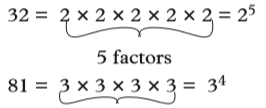The raised numeral is called an index (plural indices), power or exponent. Representing a number in this short form is known as index notation.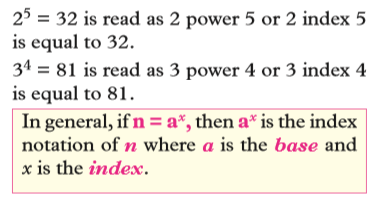For example, 16 can be factorised using a factor tree as shown below.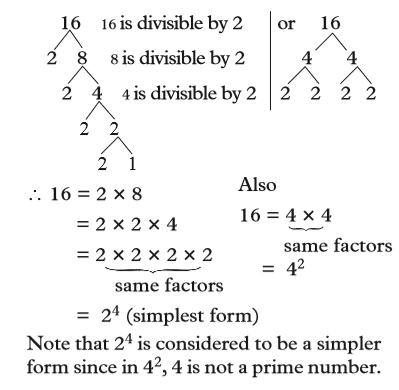Example 1.1

Write each of the following in its simplest index form.

(a) 81     (b)  96      (c)  5 × c × c × 5 × c × 5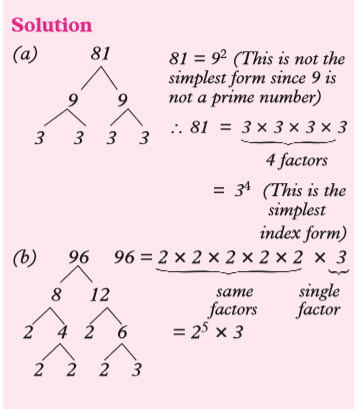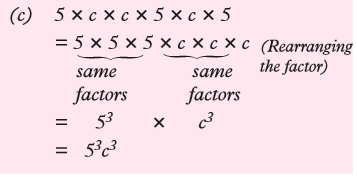Exercise 1.1

1. Write each of the following in index form using the specified base. (a) 25 (base 5)

(b) 64 (base 4)

(c) 49 (base 7)

(d) 1 000 (base 10)

2. Write each of the following in its simplest index form.

(a) 2 × a × a × a

(b) 3 × y × y

(c) h × h × h × 7 × h × 21

(d) 3 × b × b × a × b × b × b

(e) 3 × a × 3 × a × a × a

3.Write each of the following in its simplest index form.

(a) 2                   (b) 8

(c) 32                  (d) 16

(e) 64                    (f) 128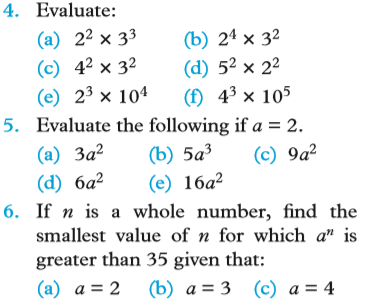7. Express 2 × 2 × 2 × 3 × 3 × 5 × 5 × 5 × 5 in index form.

8. Express 72 and 108 as products of powers of 2 and 3.

1.1.2 Properties of indices

1.1.2.1 Multiplication law of indices

Activity 1.2

1. Write the following numbers as products of two numbers where the two numbers are not equal or where one of the numbers is not one.  E.g 16 = 2 × 8

(a) 32    (b) 81

2. Write the short form of the prime products of the numbers you wrote.

3. Discuss with your partner the relationship between the index of the products and the indices of the numbers. 4. Compare your answer with other classmates.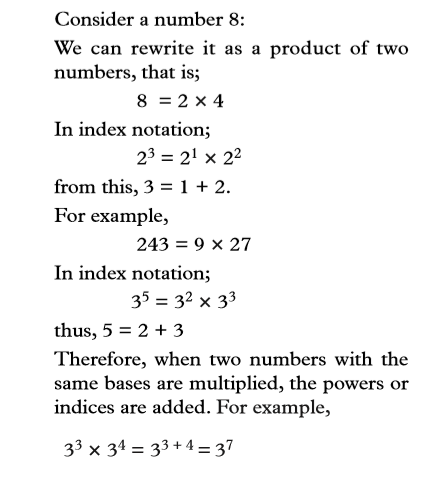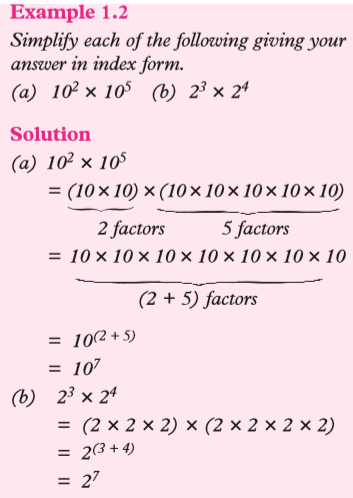Generally,

When numbers, written in index form with a common base, are multiplied, the indices are added while the base remains the same,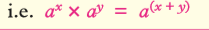If in a multiplication there is more than one letter to be multiplied, they must be multiplied separately because each represents a different value.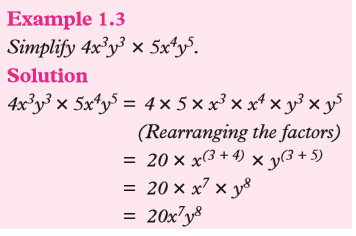Squaring an expression simply means multiplying the expression by itself.  For example,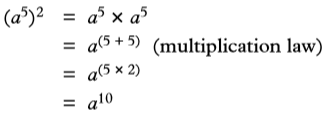Thus, in order to square an algebraic expression, square  the base and double the indices of the letters.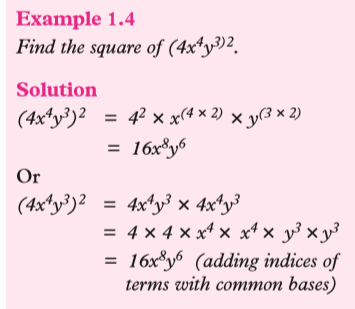1.1.2.2 Division laws of indices

Activity 1.3

1.  Write the following numbers as quotients of two numbers where the two numbers are not equal, the dividend is greater than the divisor and none of them is equal to 1.

(a) 4  (b) 3

2. Write the short form of the quotient, divisor and the dividend in index notation.

3. Discuss with your partner the relationship between the index of the quotient and that of the indices of the dividend and divisor.

4. Compare the answers with your partner.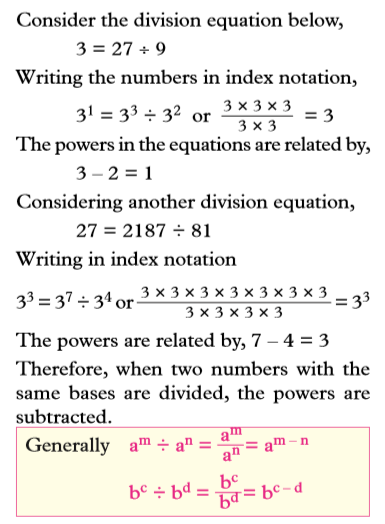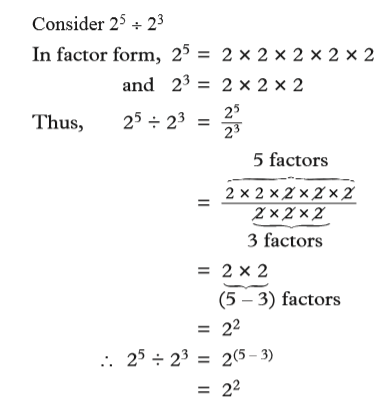In the above working, the power of 2 in the answer is the difference between indices of the numerator and that of the denominator.

Example 1.5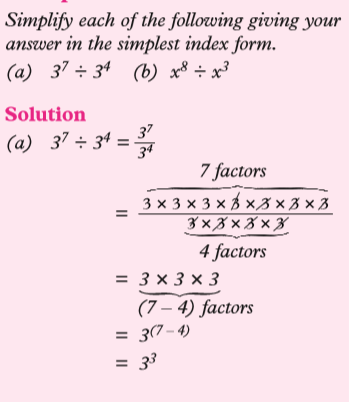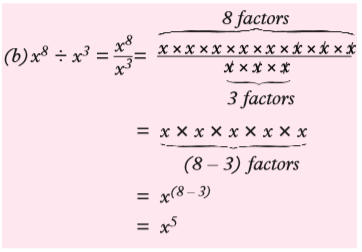If there is more than one variable to be divided, they must be divided separately as they represent different values.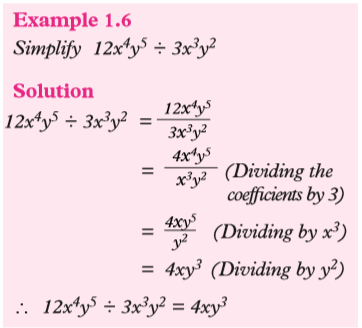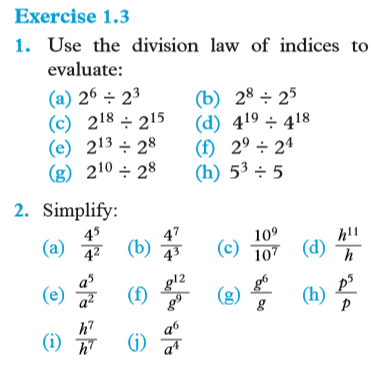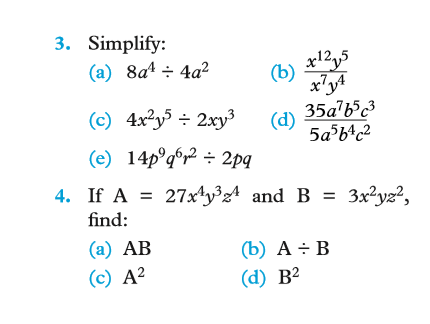1.1.2.3 Power of powers

Activity 1.4

1. Write the following numbers in index notation.

(a) 4                     (b) 27

2. Square each of the numbers.

3. Find the relationship between the indices of the squares and the solution.